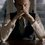# Need derivations of some formulas - 2! Help!

1. $\left( \begin{matrix} n \\ 0 \end{matrix} \right) +\left( \begin{matrix} n \\ 1 \end{matrix} \right) +\left( \begin{matrix} n \\ 2 \end{matrix} \right) +.....+\left( \begin{matrix} n \\ n \end{matrix} \right) \quad =\quad { 2 }^{ n }$

2. $\left( \begin{matrix} n \\ 0 \end{matrix} \right) +\left( \begin{matrix} n+1 \\ 1 \end{matrix} \right) +\left( \begin{matrix} n+2 \\ 2 \end{matrix} \right) +.....+\left( \begin{matrix} n+r \\ r \end{matrix} \right) \quad =\quad \left( \begin{matrix} n+r+1 \\ r \end{matrix} \right)$

3. $\left( \begin{matrix} r \\ r \end{matrix} \right) +\left( \begin{matrix} r+1 \\ r \end{matrix} \right) +\left( \begin{matrix} r+2 \\ r \end{matrix} \right) +.....+\left( \begin{matrix} n \\ r \end{matrix} \right) \quad =\quad \left( \begin{matrix} n+1 \\ r+1 \end{matrix} \right)$

4. Integrating factor - For $\frac{dy}{dx} + Py = Q$, $IF = {e}^{\int { P } dx}$

Extra Credit

If you can give me some tips for such combinatorical sums.Note by Kartik Sharma
6 years, 9 months ago

This discussion board is a place to discuss our Daily Challenges and the math and science related to those challenges. Explanations are more than just a solution — they should explain the steps and thinking strategies that you used to obtain the solution. Comments should further the discussion of math and science.

When posting on Brilliant:

• Use the emojis to react to an explanation, whether you're congratulating a job well done , or just really confused .
• Ask specific questions about the challenge or the steps in somebody's explanation. Well-posed questions can add a lot to the discussion, but posting "I don't understand!" doesn't help anyone.
• Try to contribute something new to the discussion, whether it is an extension, generalization or other idea related to the challenge.

MarkdownAppears as
*italics* or _italics_ italics
**bold** or __bold__ bold
- bulleted- list
• bulleted
• list
1. numbered2. list
1. numbered
2. list
Note: you must add a full line of space before and after lists for them to show up correctly
paragraph 1paragraph 2

paragraph 1

paragraph 2

[example link](https://brilliant.org)example link
> This is a quote
This is a quote
    # I indented these lines
# 4 spaces, and now they show
# up as a code block.

print "hello world"
# I indented these lines
# 4 spaces, and now they show
# up as a code block.

print "hello world"
MathAppears as
Remember to wrap math in $$ ... $$ or $ ... $ to ensure proper formatting.
2 \times 3 $2 \times 3$
2^{34} $2^{34}$
a_{i-1} $a_{i-1}$
\frac{2}{3} $\frac{2}{3}$
\sqrt{2} $\sqrt{2}$
\sum_{i=1}^3 $\sum_{i=1}^3$
\sin \theta $\sin \theta$
\boxed{123} $\boxed{123}$

Sort by:

For - 1) ( Although Megh aleready post it But here one another approach for this )

Let P = Total Number's of ways of selecting n things (Say shoes ) in all possible ways

So Obviously , LHS = P

and also there is two possibility for one shoe for selection , i.e either it will be selected or not .

So all possible ways is " P " $P\quad =\quad 2\times 2\times 2\times 2\quad .\quad .\quad .\quad .\quad .\quad .\quad 2\quad =\quad { 2 }^{ n }\quad$

- 6 years, 9 months ago

Another method for 3. ( but i think the usual is better)

Let any r of n + r + 1 objects given. let it be $a_{1} , a_{2} ............ , a_{r}$

Case 1 - it does not contain $a_{1}$ , it will happen in $\binom{n + r}{r}$ ways for the r things to choosen from the remaining n + r things.

Case 2 - it contains $a_{1}$ but does not contain $a_{2}$ . This will happen in $\binom{n + r - }{ r - 1}$ ways (we have chossen $a_{1}$ and not $a_{2}$ , we have only n + r - 1 things to choose from and we need only r - 1)

Case 3 - it contains $a_{1} , a_{2}$ and not $a_{3}$ , can happen in $\binom{n + r - 2}{r - 2}$ ways(same reasoning)

Case 4 , case 5 ...... etc

Case r - it contains $a_{1} , a_{2} , ...... a_{r - 1}$ but not $a_{r}$ , can happen in $\binom{n + 1}{1}$ ways.

case r +1 - it contains all things , can happen in $\binom{n}{0}$ways .

Thus-

$\binom{n}{0} + \binom{n + 1}{1} + ............. + \binom{n + r}{r} = \binom{n + r + 1}{r}$

- 6 years, 9 months ago

1.here (x, y) = (1 , 1)

thus it is $( 1 + 1)^{n} = 2^{n}$

1. $\binom{n + r + 1}{r} = \binom{n + r}{r} + \binom{n + r}{r - 1}$

$\binom{n + r }{r - 1} = \binom{n + r - 1}{r - 1} + \binom{n + r - 1}{r - 2}$

$\binom{n + r - 1}{r - 2} = \binom{n + r - 2}{r - 2 } + \binom{n + r - 3}{r - 3}$

.

.

.

$\binom{n+3}{2} = \binom{n + 2}{2} + \binom{n + 2}{1}$

$\binom{n+2}{1} = \binom{n + 1}{1} + \binom{n + 1}{0}$ $= \binom{n + 1}{1} + \binom{n}{0}$

$\binom{n}{0} + \binom{n + 1}{1} + ............. + \binom{n + r}{r} = \binom{n + r + 1}{r}$

I think now you can derive the third @Kartik Sharma

Sorry i don't know the fourth

- 6 years, 9 months ago

Yeah, I thought of the same! Try the others!

- 6 years, 9 months ago

Use fundamental formulas to derive these kinds of sums @Kartik Sharma

- 6 years, 9 months ago